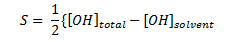# Calcium Hydroxide

The solubility of calcium hydroxide (Ca(OH)2) is given as:The solubility is a factor of total hydroxide ion in the solution and the solvent hydroxide concentration. The solvent is the liquid in which the calcium hydroxide is dissolved. Which of the following would increase the solubility of calcium hydroxide?Loading ...

Tags: ,